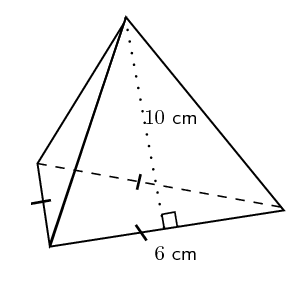# Triangular Pyramid Formula

When you think about a Pyramid, the first thing that comes to your mind is the Great Pyramids of Egypt. If you observe the shape of the Great Pyramid of Egypt, then you will notice that the pyramid has a base, that is square shaped. Hence, those pyramids are called Square Pyramid. Similarly, in the triangular pyramid the base of the same is triangular.

When a base is constructed to connect to the apex, a pyramid is formed. A pyramid that had a triangular base is a triangular pyramid. It is also known as the tetrahedron which has equilateral triangles for each of its faces. The illustration below will make it clear what the triangular pyramid looks like.There are majorly two formulas for triangular pyramid:

$\large Volume\;of\;a\;triangular\;pyramid=\frac{1}{3}Base\;Area\times Height$

Surface area of triangular pyramid = A + 3a

where A is the base area and ‘a’ is the area of one of pyramid’s faces.

### Examples of Triangular Pyramid

Question 1: Find the volume of a triangular pyramid when base area is

$$\begin{array}{l}9\;cm^{2}\end{array}$$
and height is 4 cm ?

Solution:
Given,
Base area =

$$\begin{array}{l}9\;cm^{2}\end{array}$$
Height = 4 cm

As we know,

$$\begin{array}{l}Volume\;of\;a\;triangular\;pyramid=\frac{1}{3}\;Base\;Area\times Height\end{array}$$

According to the formula:
$$\begin{array}{l}\frac{1}{3}\times9\times4=12\,cm^{2}\end{array}$$